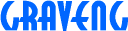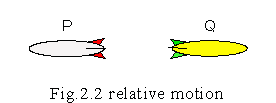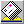2.2 Absolutely velocity

Suppose two spaceships 'P' and 'Q' are floating in the space as not exist influence of the gravity from others. And only 'P' and 'Q' exist in the space, an observer in 'P' can see only 'Q', opposite, an observer in 'Q' can see only 'P', the each observer can not see other object. At the beginning, 'P' and 'Q' are separated in fixed short distance. When relative velocity between 'P' and 'Q' is zero. At the next, one of 'P' or 'Q' is accelerated by rocket engine. Which one accelerated can the each observer get to know?Observing from 'P', it seems that 'Q' goes away in relative acceleration observed. Quite similarly, observing from 'Q', 'P' goes away in same acceleration. Person like Mach, Poincare, Einstein probably say about this experiment as;

"We have always decided position or velocity of object by comparing others object. In this case, comparable object for 'P' is only 'Q', for 'Q' is only 'P'. Measured relative acceleration is the quite same as 'P' and 'Q' except contrary direction. If did based on 'P', 'Q' was accelerated. Based on 'Q', 'P' was. Therefore, because it is observed in the same way at all, there is no way of distinguishing which was accelerated, and the way is not exist."

What it thinks about like this is really right if the knowledge of the existent physics is used. It is because the thing said as absolute acceleration and absolute velocity is denied by the physicist's general opinion. But, you would probably already have noticed that the way exist. "It can be get to know which was accelerated for the observer who was in 'P' or 'Q'. When it gets on an accelerated car, it is felt that a body is pressed against the seat. There may be such force in spaceships too, the observers can know whether their spaceship was accelerated or not." It is positive. If an acceleration meter is installed in the spaceship, they can get to know how much accelerated and which direction to go precisely unless see outside of the spaceship.

You might think that such thought had been said first by Einstein. He certainly said like that pulling elevator which floating in the zero gravity space, an apple in the elevator is falling. However, the experiment and 'relative motion' contradict fundamentally. What on based relative acceleration is the acceleration occurred by pulling elevator? There is not exist such object and not necessary. The acceleration is not relative acceleration.

If conception of velocity was only relative velocity, acceleration which function of velocity would be also only relative quantity as relative acceleration. However, there was no way of examining which of 'P' or 'Q' moved using conception of the relative acceleration. but using acceleration meter, there is the way exist. Therefore, obviously it isn't the same as the quantity of the relative acceleration and the value of the acceleration meter in the spaceship. It is natural that they can't be defined as the same quantity.

Using existent physics, somewhere based position was necessary for measuring of velocity or displacement against object. Acceleration can be measured by the way of acceleration mater useless to compare other based position in the space. The acceleration of the mater is not chased on coordinates, can measure acceleration by installed in moving object. Accelerating 'P' Installed the acceleration meter using non-gravity force, It is experientially known that the value of acceleration meter is the same as relative acceleration measured use of the origin of a coordinates axis set on 'Q' position. We can integral calculate velocity or displacement by value of the meter easily. To distinguish with relative quantity, let's decide the quantity to be called 'inertia acceleration', 'inertia velocity' and 'inertia displacement'

Suppose that 'Q' was not accelerated, amount value of acceleration meter in 'Q' was zero, that also inertia acceleration and inertia velocity was zero. looking at 'Q' from 'P', 'Q' was moving with relative velocity. We can find out precisely the difference 'P' and 'Q' using inertia acceleration or inertia velocity.

It isn't supposed to be able to be insisted that there are some differences about the condition of 'P' and 'Q' using relative velocity which only shows the relative position. Relative velocity changes in the way of taking a coordinates, and can take it free. It is not the directly relation between quantity of relative velocity and condition of moving object.

Inertia velocity is not necessary to compare with others at the measuring. The quantity which is not compared with others is considered absolute quantity. Absolute quantity is the quantity which can show its condition. We can be considered that it is the physical different condition with 'P' and 'Q' using the absolute quantity. If we use the inertia velocity, could express, no contradict, that moving is train, but station.

The force moving acceleration meter is explained using 'apparent force' like ghost in general physics. They would say that "it is apparent" with keeping point at the acceleration meter actually working. This strange word must be introduced in the physics that the reason is the vagueness of the recognition about the velocity. Next chapter, we will bust the ghost using new weapon as inertia velocity.

Updated 29/Mar/1997redsky@graveng.jp